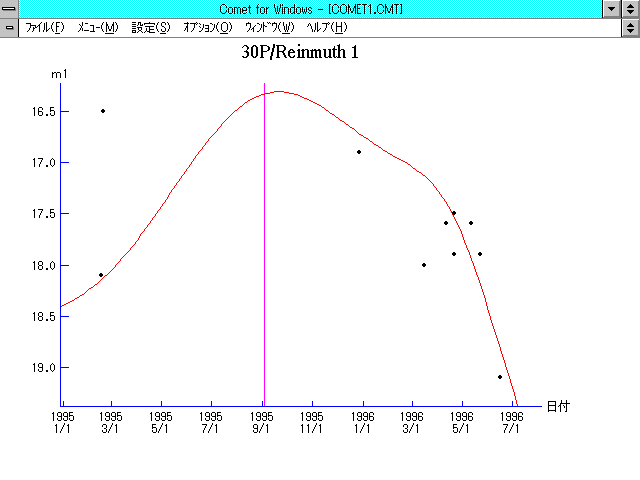# \$B%i%\$%s%`!<%HBh#1WB@1(B

30P/Reinmuth 1 (1995)###\$B50F;MWAG(B

```   The following improved orbital elements by Kenji Muraoka,
are from 183 observations 1957 to 1996, including 9 Planets,
Moon and 5 minor planets perturbations and non-gravitational
effect of style II. The mean residual is +/- 0.90 arc seconds.

Epoch  =  1995 Aug. 31.0  TT       JDT = 2449960.5
T  =  1995 Sept. 3.32895       +/- 0.00044 (m.e.) TT
Peri. =   13.28865                +/- 0.00022
Node  =  119.74131                +/- 0.00017   (2000.0)
Incl. =    8.12912                +/- 0.00003
q  =    1.8736020              +/- 0.0000017 AU
e  =    0.5024914              +/- 0.0000003
a  =    3.7659689              +/- 0.0000011 AU
n  =    0.13486181             +/- 0.00000006
P  =    7.308                  +/- 0.0000033  years
A1  =   +0.254                  +/- 0.021
A2  =   -0.01541                +/- 0.00025
```

###\$B@1?^(B1994\$BG/(B10\$B7n(B 5\$BF|!A(B1995\$BG/(B 9\$B7n(B10\$BF|(B1995\$BG/(B 7\$B7n(B12\$BF|!A(B1996\$BG/(B 6\$B7n(B16\$BF|(B

###\$B8wEYJQ2=(B

```        m1 = 10.0 + 5 log\$B&\$(B + 15.0 log r
```##### \$B50F;MWAG\$OB<2,7r<#;a\$N7W;;\$K\$h\$k\$b\$N\$G\$9!#(B \$B@1?^\$O(B StellaNavigator Ver.2.0 for Windows (\$B%"%9%H%m%"!<%D(B \$BJTCx(B / \$B%"%9%-!<=PHG6I4)(B) \$B\$G:n@.\$7\$?\$b\$N\$G\$9!#(B \$B8wEY%0%i%U\$O(BComet for Windows\$B\$G:n@.\$7\$?\$b\$N\$G\$9!#(B